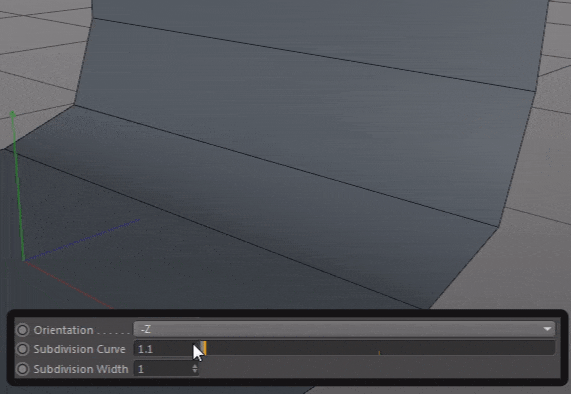# Solved Linearize an exponential function

Hi,
I have created an user data slider (with percent unit) to controlling subdivisions number of a spline (spline with an Adaptive interpolation). So I have used a MapRange function to convert a percentage to a degree but this dosen't returnning a natural result. because the number of an intermediate subdivision points is increasing exponentially.
I want to know how to fit an exponential number of intermediate points to a linear.

``````        SplineObject *sp = SplineObject::Alloc(4, SPLINETYPE_BEZIER); // create a spline with 3 verts
Vector *gp = sp->GetPointW();

gp = Vector(0, 0, 0);
gp = Vector(0, 0, 900);
gp = Vector(0, 250, 1200);
gp = Vector(0, 900, 1200);

sp->GetDataInstance()->SetBool(SPLINEOBJECT_CLOSED, false);

Float value = data->GetFloat(BSK_OBJECT_CURVE_SUB);
Float min_input = 0;
Float max_input = 1;
Float min_output = 90;
Float max_output = 1;

Float inrange = max_input - min_input;
if (CompareFloatTolerant(inrange, 0.0)) value = 0.0;
else value = (value - min_input) / inrange;
curve_sub = DegToRad(min_output + (max_output - min_output) * value);

sp->SetParameter(SPLINEOBJECT_ANGLE, curve_sub, DESCFLAGS_SET_0);
``````

@r_gigante
Finally I found the solution to linearize the subdivision expansion on Adaptive spline interpolation on the angle deviation.

I just replaced the line :

``````radVal = c4d.utils.DegToRad(90 * (1.0 - val)
``````

By :

``````radVal = math.pow(val, -0.85)
``````

I changed also the slider user data unit to REAL
if I want to keep it with a PERCENT unit I just multiply the value by 100

``````radVal = math.pow(val*100, -0.85)
``````

Here is demo:Hi mfersaoui, thanks for reaching out us.

With regard to your issue I've a few notes:

• the Adaptive interpolation is by definition strictly dependent on the shape of the spline with regard to the tessellation of the curve: the curve will be tessellated more where the curvature is larger and this generates evidently non-uniform segments distribution - check here for further notes -;
• standing on the code presented the `in_range` variable is always 1.0 which makes this
``````if (CompareFloatTolerant(inrange, 0.0)) value = 0.0;
else value = (value - min_input) / inrange;
``````

pretty useless.

I would recommend, assuming that the adaptive strategy is the one you want to keep to consider ( sorry for the python code, but porting should be trivial)

``````        # swap the max/min values of the slider
val = op[c4d.ID_USERDATA, 1]
# set the interpolation method
interp = 3
# allocate the spline
spline = c4d.SplineObject(4, c4d.SPLINETYPE_BSPLINE)
# set interpolation method
spline[c4d.SPLINEOBJECT_INTERPOLATION] = interp
# switch according to interpolation method
if interp == 2: # uniform
# set the max number of segs
maxSegs = 100
spline[c4d.SPLINEOBJECT_SUB] = int(maxSegs * val)
if interp == 3: # adaptive
# get the rad representation of the slider's value
# set the adaptive angle value
# set the points
point = spline.SetAllPoints((c4d.Vector(0,0,0),c4d.Vector(0,0,900),c4d.Vector(0,900,900), c4d.Vector(0,900,0)))
# return the spline
return spline
``````

If instead you'd like to linearly increase the subdivision, I warmly recommend to switch to the uniform method and you'll see that a linear behavior is established

Best, R

@r_gigante Thank you for the detailed reply,

Sorry, I didn't put the last part of my code in my question above. I will mention that I want to set a tangent on the points 2 and 3, here is the last part of my code in python:

``````curve_offset = op[c4d.BSK_OBJ_CURVE_OFFSET] # correspond to the value "250" in my above code
spline.SetTangent(1, Vector(0, 0, 0), Vector(0, 0, curve_offset * 0.5))
spline.SetTangent(2, Vector(0, -curve_offset * 0.5, 0), Vector(0, 0, 0))
``````

So, I try to add the lines above on your code but this returning this error message:

``````IndexError: tangent index out of range
``````

Thanks Again.

@r_gigante
Finally I found the solution to linearize the subdivision expansion on Adaptive spline interpolation on the angle deviation.

I just replaced the line :

``````radVal = c4d.utils.DegToRad(90 * (1.0 - val)
``````

By :

``````radVal = math.pow(val, -0.85)
``````

I changed also the slider user data unit to REAL
if I want to keep it with a PERCENT unit I just multiply the value by 100

``````radVal = math.pow(val*100, -0.85)
``````

Here is demo: